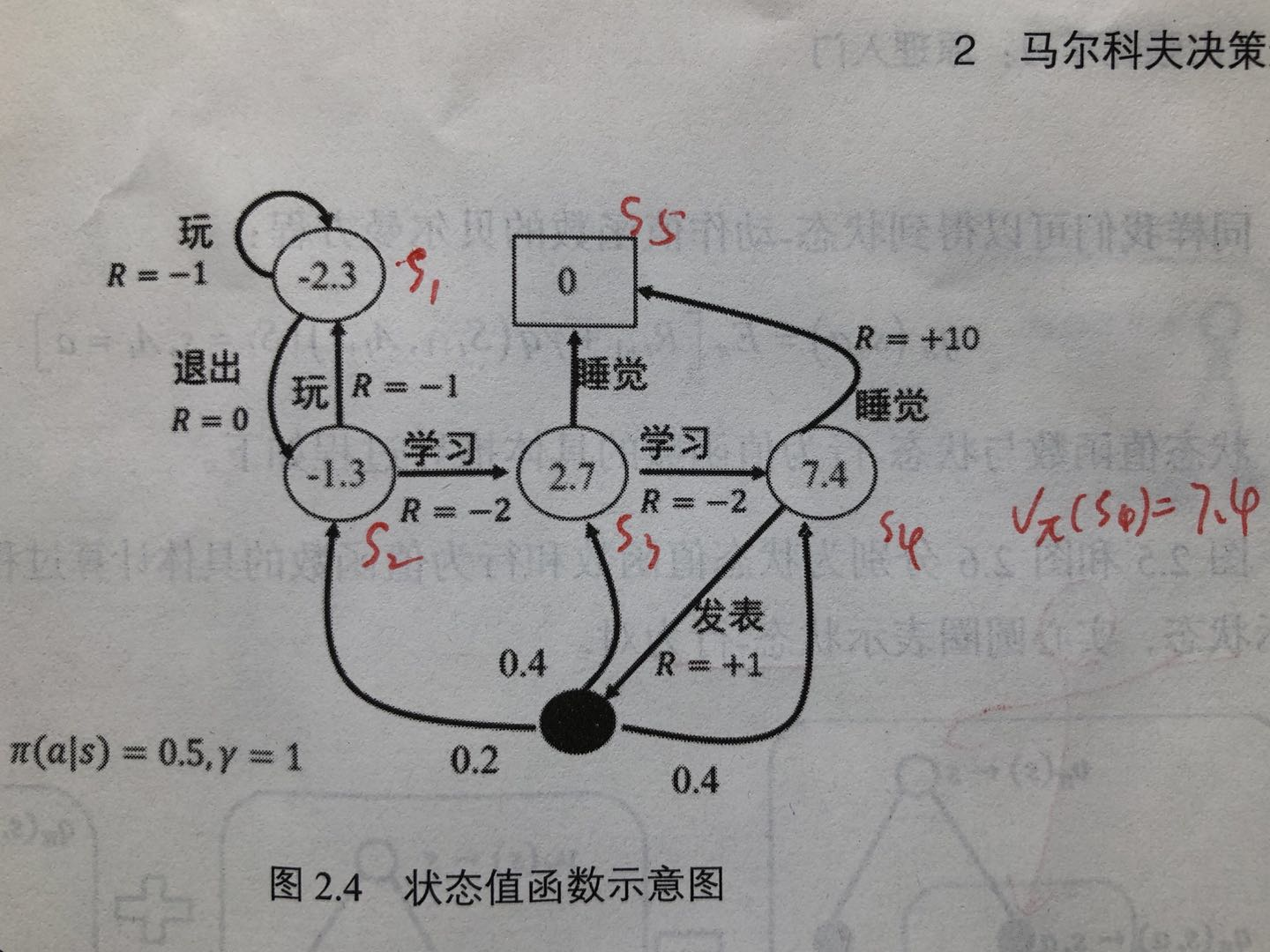To Top

## 1. 马尔科夫决策过程理论

### 1.2 马尔科夫过程

$P=\begin{bmatrix} P_{11} & ... & P_{1n} \\ \vdots & \vdots & \vdots \\ P_{n1} & ... & P_{nn} \end{bmatrix}$

### 1.3 马尔科夫决策过程

• $$S$$为有限的状态集
• $$A$$为有限的动作集
• $$P$$为状态转移概率
• $$R$$为回报函数
• $$\gamma$$折扣因子，用于计算累积回报

$\pi (a|s)=p[A_i=a|S_t=s]$

• 强化学习的策略往往是随机策略。可以将探索耦合到采样的过程中。探索，指机器人尝试其他动作以便找到更好的策略
• 实际应用中，存在各种噪声，这些噪声大都服从正态分布，可以用概率的知识去掉噪声

$G_t=R_{t+1}+\gamma R_{t+2}+...=\sum _{k=0}^{\infty }\gamma ^kR_{i+k+1}$

### 1.4 状态值函数与状态-行为值函数

$\upsilon _{\pi}(s)=E_{\pi}[\sum _{k=0}^{\infty }\gamma ^kR_{t+k+1}|S_t=s]$

$q_{\pi}(s,a)=E_{\pi}[\sum _{k=0}^{\infty}\gamma ^kR_{t+k+1}|S_t=s,A_t=a]$

### 1.5 状态值函数与状态-行为值函数的贝尔曼方程

$\begin{split} \\\upsilon(S_t)&=\upsilon(s)=E[G_t|S_t=s] \\&=E[R_{t+1}+\gamma R_{t+2}+...|S_t=s] \\&=E[R_{t+1}+\gamma (R_{t+2}+\gamma R_{t+3}+...)|S_t=s] \\&=E[R_{t+1}+\gamma G_{t+1}|S_t=s] \\&=E[R_{t+1}+\gamma \upsilon(S_{t+1})|S_t=s] \end{split}$

$\begin{split} \\\upsilon(S_t)&=E_{S_t,S_{t+1},...}(R_{t+1}+\gamma G_{t+1}|S_t=s) \\&=E_{S_t}(R_{t+1})+\gamma E_{S_{t+1},...}(G_{t+1}) \\&=E_{S_t}(R_{t+1}+\gamma \upsilon(S_{t+1})) \\&=E(R_{t+1}+\gamma\upsilon(S_{t+1})) \end{split}$

$q_{\pi}(s,a)=E_{\pi}[R_{t+1}+\gamma q(S_{t+1},A_{t+1})|S_t=s,A_t=a]$

$\upsilon_{\pi}(s)=\sum_{a\in A}\pi(a|s)q_{\pi}(s,a)$

$q_{\pi}(s,a)=R^a_{s}+\gamma \sum _{s'}P^a_{ss'}\upsilon _{\pi}(s')$

$\upsilon_{\pi}(s)=\sum_{a\in A}\pi(a|s)(R^a_{s}+\gamma \sum_{s'\in S}P^a_{ss'}\upsilon_{\pi}(s'))$

$\upsilon_{\pi}(s')=\sum _{a'\in A}\pi(a'|s')q_{\pi}(s',a')$

$q_{\pi}(s,a)=R^a_{s}+\gamma \sum_{s'\in S}P^a_{ss'}\sum _{a'\in A}\pi(a'|s')q_{\pi}(s',a')$$\begin{split} \\\upsilon (s_4)&=\pi(睡觉|s_4)R^{睡觉}_{s_4s_5}+\pi(发表|s_4)(R^{发表}_{s_4s_3}+\gamma (P^{发表}_{s_4s_2}\upsilon (s_2)+P^{发表}_{s_4s_3}\upsilon (s_3)+P^{发表}_{s_4s_4}\upsilon (s_4))) \\&=0.5*10+0.5*(1+1*(0.2*(-1.3)+0.4*2.7+0.4*7.4)) \\&=7.39 \end{split}$

### 1.6 最优状态值函数与最优状态-行为值函数及其贝尔曼最优方程

$\upsilon ^*(s)=\underset{a}{max}R^a_{s}+\gamma \sum _{s'\in S}P^a_{ss'}\upsilon ^*(s')$

$q^*(s,a)=R^a_{s}+\gamma \sum _{s'\in S}P^a_{ss'}\underset{a'}{max}q^*(s',a')$

$\pi_*(a|s)=\left\{\begin{matrix} 1 &if\ a=\underset{a\in A}{argmax}q_*(s,a) \\ 0 & otherwise \end{matrix}\right.$

### 1.7 强化学习形式化描述

• $$S$$状态集
• $$A$$动作集
• $$P:S\times A\times S\rightarrow R$$转移概率
• $$r:S\times A\rightarrow [-R_{max},R_{max}]$$立即回报函数
• $$\rho_0:S\rightarrow R$$初始状态分布
• $$\gamma \in [0,1]$$折扣因子
• $$T$$水平范围（即步数）

$$\tau$$为一个轨迹序列$$\tau =(s_0,a_0,s_1,a_1,...)$$ $$R=\sum _{t=0}^T\gamma ^tr_t$$累积回报

$\underset{\pi}{max}\int R(\tau )p_{\pi}(\tau )d\tau$

## 2. MDP中的概率学基础

### 2.1 期望与方差

#### 期望

• 离散型随机变量的期望：

$E_{x\sim P}[f(x)]=\sum _xP(x)f(x)$

• 连续型随机变量的期望通过积分求得：

$E_{x\sim P}[f(x)]=\int p(x)f(x)dx$

$E_x(\alpha f(x)+\beta g(x))=\alpha E_x[f(x)]+\beta E_x[g(x)]$

#### 方差

$Var(f(x))=E[(f(x)-E[f(x)])^2]$

### 2.2 随机策略

#### 2.2.1 贪婪策略

$\pi_*(a|s)=\left\{\begin{matrix} 1 &if\ a=\underset{a\in A}{argmax}q_*(s,a) \\ 0 & otherwise \end{matrix}\right.$

#### 2.2.2 $$\varepsilon -greedy$$策略

$\pi (a|s)=\left\{\begin{matrix} 1-\varepsilon + \frac{\varepsilon}{|A(s)|} &if\ a=\underset{a\in A}{argmax}q_*(s,a) \\ \frac{\varepsilon}{|A(s)|} & otherwise \end{matrix}\right.$ $$\varepsilon -greedy$$策略是强化学习最基本最常用随机策略。该策略平衡了e&e，也就是利用(exploitation)探索(exploration)，其中选取动作值函数最大的部分为利用，其他非最优动作仍然有概率的部分为探索部分。

#### 2.2.3 高斯策略

$\pi_{\theta}=\mu_{\theta}+\varepsilon,\varepsilon \sim N(0,\sigma ^2)$

#### 2.2.4 玻尔兹曼分布

$\pi(a|s,\theta)=\frac{exp(Q(s,a,\theta))}{\sum_bexp(h(s,b,\theta))}$

## 3. 基于gym的MDP实例

https://github.com/daiwk/reinforcement-learning-code/blob/master/grid_mdp.py

comment here..